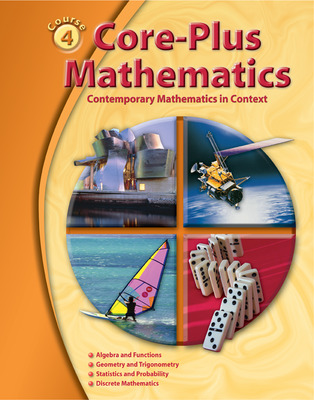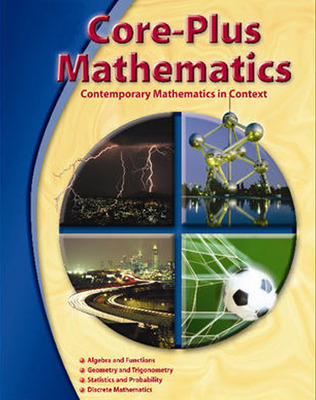# Core-Plus Mathematics: Course 4: Preparation for Calculus

Funded by the National Science Foundation, Core-Plus Mathematics is a four-year, international-like high school mathematics program that aligns well with the Common Core State Standards for Mathematics, including the Standards of Mathematical Practice.

Course 4 continues the preparation of students for college mathematics, with a focus on preparation for calculus. The text is designed so that students learn important mathematics and mathematical habits of mind through inquiry and problem-based investigations featuring realistic contexts.

Core-Plus Mathematics provides extensive teacher support and a suite of free available mathematical software tools• Development of mathematics as an active science of patterns involving quantity and change, shape and motion, data and chance, and counting and algorithms
• Courses organized around interwoven strands of
• algebra and functions
• geometry and trigonometry
• statistics and probability
• discrete mathematics
• Mathematical strands developed in coherent, focused units connected by fundamental unifying ideas
• Mathematics developed in context with an emphasis on applications and mathematical modeling
• Student-centered investigations promote active learning through problem solving
• Full and appropriate use of technology tools, including new CPMP-Tools® software for each course

## Program Components

•Core-Plus Course 4 Online Teacher's Guide (1-Year Subscription)
•Implementing the Core-Plus Mathematics Curriculum
Sort by: